Select Page

## Completing Part VI, Section D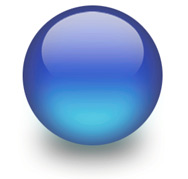All the numbers we have been dealing with concerning the Torah’s first verse and its cubic nature, form a circle. They all circle and cycle back on each other, starting with 173, as the sum of the small gematria of the 42-Letter Name. There was 37 and 73 and 127 and 137 and they are all prime numbers. Then there were the offshoots of these numbers like 27 = 128 and 64 with 7 being a prime number and 64 already being a cube.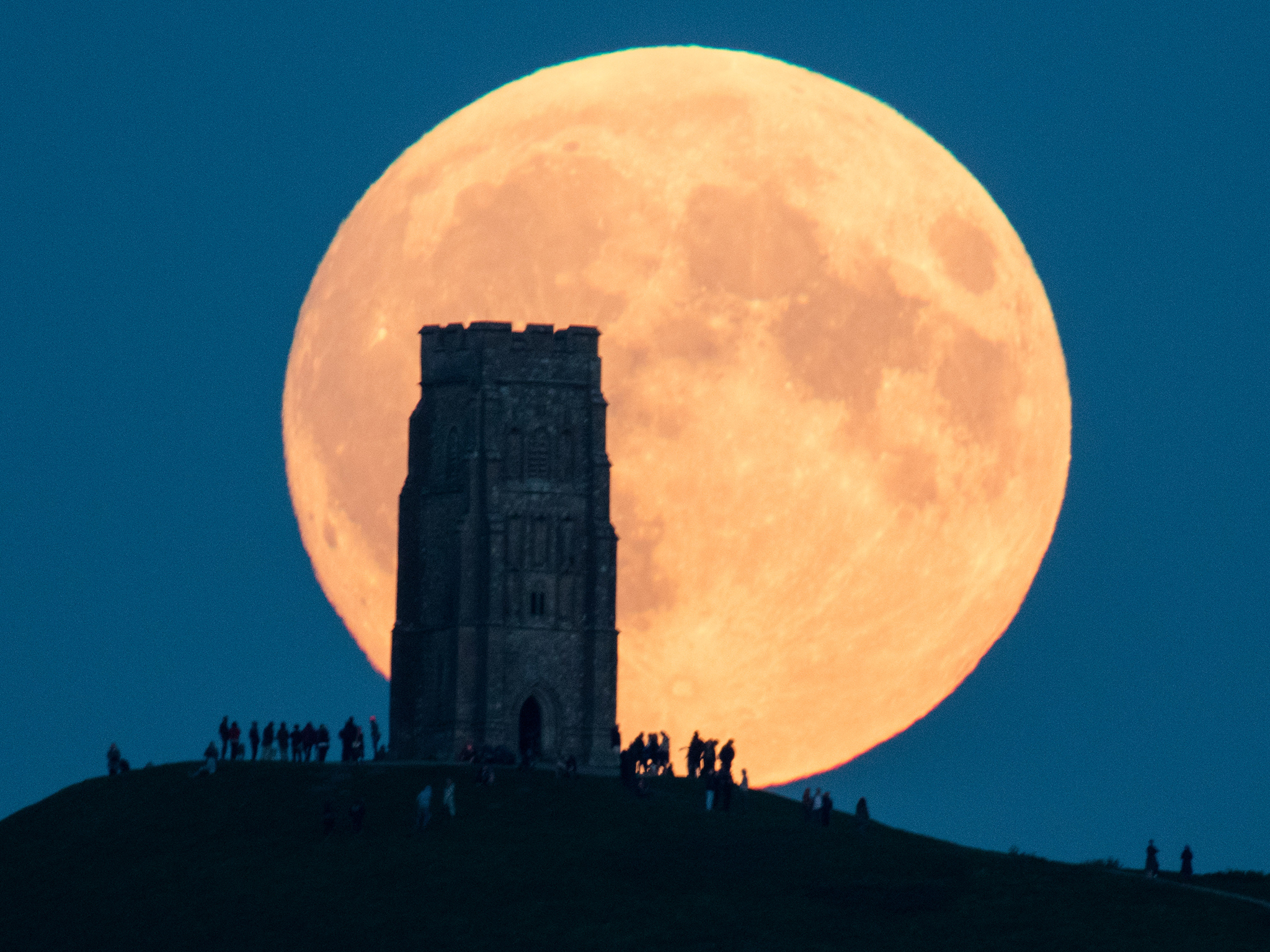We begin with 173, the 40th prime. Add to it (37 and 73), the (12th and 21st) primes respectively and we realize that not only is (37 x 73) equal to 2701, the value of the entire first verse, but (12 + 21) is equal to 33, as in the 33 letters of the first 8 words. Next, we realize that their primal positions 40 + 12 + 21 are equal to 73, as in the sum of the integers from 1 – 73 that equal 2701. The circle continues as 137 is the 33rd prime number. And the total number of possible combinations for the 7 first words, 127, is the 31st prime. Together, (33 + 31) = 64, as in the 64 cubic times each of the 7 words could be individually combined. Moreover, just as 73 + 64 = 137, so naturally do the primal locations (40 + 12 + 21) + (33 + 31) = 137.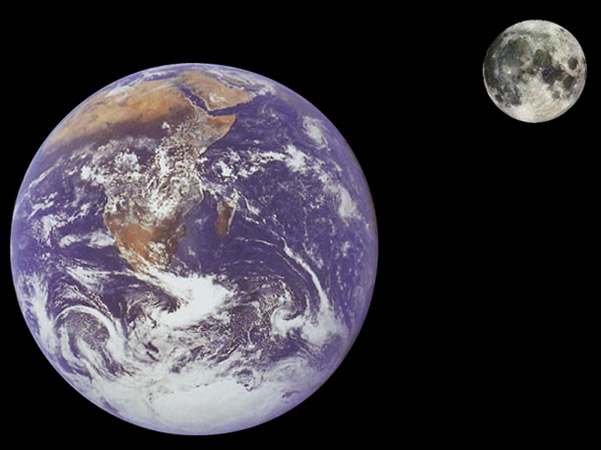Then, while (173 + 37 + 73) = 283, the 61st prime, (173 + 37 + 73 + 137 + 127) = 547, the 101st prime.  And 101 is the 26th prime, as in the value of the Tetragrammaton (יהוה), with (101 + 26) being equal to 127, the 7th Mersenne Prime and 31st overall Prime once again.
Let us press “pause” for a second, because the Name Israel is exactly found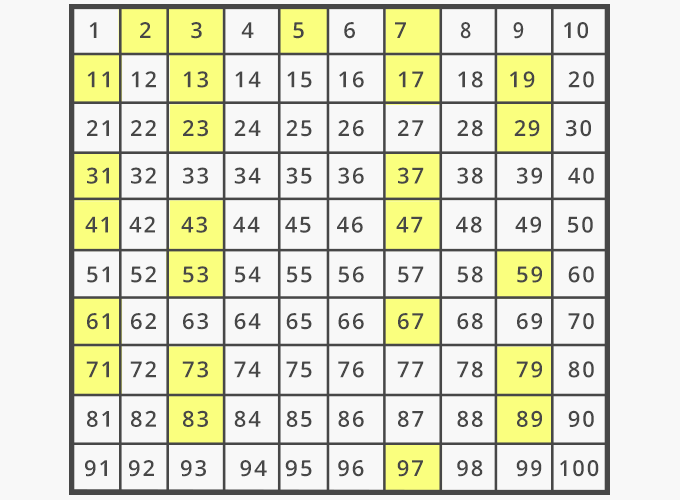547 times in the Torah, and its numerical value is 541, the 100th prime, the One that precedes 547, the sum of our Prime core circle of 5. Are the primes, marking places in a cosmic matrix, or are they discreet irreducible prime energy levels, or both? Should we be looking at the prime numbers as the elements in our periodic table perhaps? Are we looking at our universe in the right way?  Or is this extremely sophisticated design of the Torah illustrating to us that there is also a much more sophisticated design to the universe as well?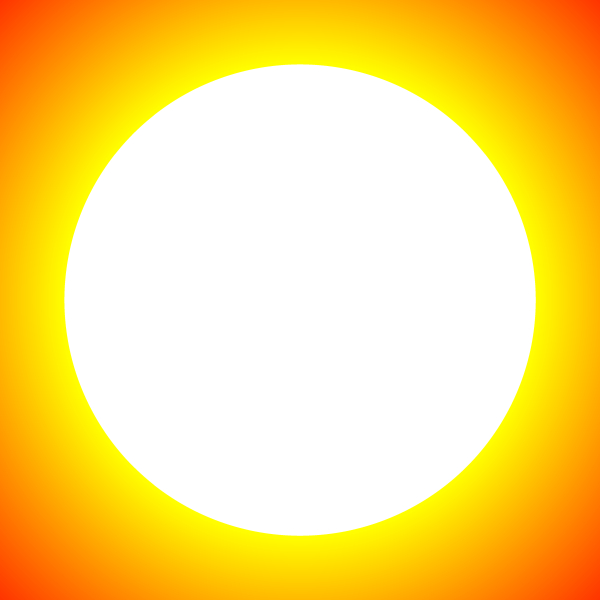Does it mean anything that the value of the Name Israel, 541 is found 625 times in the Torah, matching the square root of the 3 main Torah elements, 625, found 9 (3 x 3) times in the Torah. Or that the Name Israel is exactly found 547 times in the Torah, and its numerical value is 541, the 100th prime and that 547 + 541 + 1 = 332?  Or that 547 is both the sum of out 5 core Prime Genesis Numbers (173 + 37 + 73 + 137 + 127) = 547, and the 101st prime, with 101 – 100 = 1? And since 101 is well known to the kabbalists as the gematria of “Michael,” the archangel protector of Israel, is it possible they were aware of this connection on some level and that Michael was a specific energetic archetype uniquely associated with Israel? In the same way, is the archangel Metatron of numerical value 314 a specific energetic archetype uniquely associated with knowledge? Or the archangel Raziel of numerical value 248, could he be a specific energetic archetype uniquely associated with secret knowledge? There are only 7 of them. It is not as if their names of numerical equivalents were chosen randomly.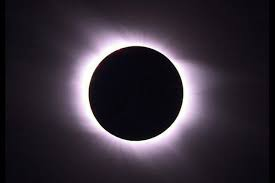We can add to that list of select core primes, the sum of the unique Primeth Recurrence series through 127 and get 179, the 41st Prime.  And while (173 and 137) have primal locations of (40 and 33) respectively, which sum to 73, the sum of (173 + 137) + (40 + 33) = 383, the 76th prime and 76 is the ordinal value, as we know, of the Torah’s first word.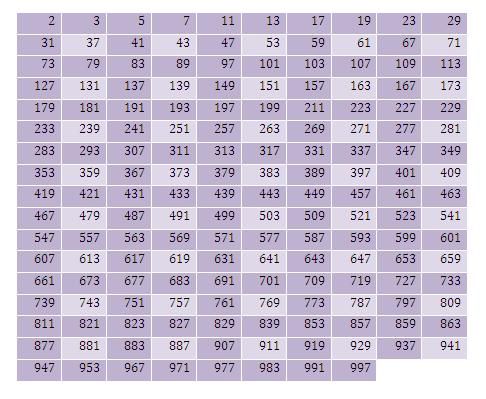What the rest of our inner circle of primes have in common is found in their locations: 73 is the 21st prime; 127 is the 31st one; 179 is the 41st one; 283 is the 61st one; and 547 is the 101st one. If the primes were laid out as a 10 by x matrix, they would all line up in the first column (21st, 31st, 41st, 61st, 101st).  And along with our discussion of the negative or null space within the first verse, we see that 173 – (37 + 73) = 63, as in the 63 null space cells for each of the 127 combinations. And considering 173 is the 40th prime and 37 and 73 are the 12th and 21st primes respectively, we get 40 – (12 + 21) = 7, which is the 4th prime, and 7 x 4 = 28, while the 28th prime is 107, and the sum of the integers from 1-107 is 5778.
As far as complementary numbers to this prime set, (173 -73) = 100; and (137 – 37) = 100; then there is 27 = 128; and (128 – 127) = 1; and (128 – 28 letters) = 100, which leads to 107 – 7 = 100.
Finally, 37 + 73 + 137 + 173 = 420. We could add 4 for the 4 elements in the equation, as all the preeminent kabbalists would, to get 424, or just leave it as 10 x 42.
Time to stop the presses again. Remember how (173 + 137 + 127 + 73 + 37) = 547, the 101st prime and Israel was found 547 times in the Torah, well…. The numerical value 173 is found 13 times in the Torah; that of 137 is found 57 times; that of 127 is incredibly found 64 times, considering their relationship in the combinations of the 7 words; that of 73 is found 89 times; and that of 37 is found 555 times. Collectively, the 5 core primes total 778. Need we say more.

## Where is the Pilot’s Seat to this Ship?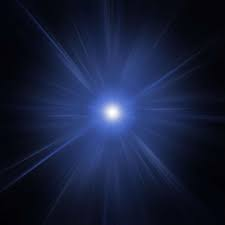If the first verse is a command module of sorts, where is the pilot’s seat? Another question could be who is the pilot? As with any treasure hunt, we need to follow the clues. There are six visible alefs(א) in the Torah’s first verse for a total name value of 6 x 111 or 666. They occur in every word of the verse except the 5th word, H’Shamayim (השמים), meaning “The Heavens.” The three central letters of H’Shamayimשמים) are the same 3 letters in Moshiach (משיח). If we substitute Moshiach (משיח) of numerical value 358 for H’Shamayim (השמים) of numerical value 395 the difference is 37, meaning a 37-unit reduction in the value of the first verse from 2701 to 2664. While 37 is telling as one of our Prime Core Numbers, and 64 is one of our main cubic numbers also associated with that verse, 2664 is exactly 4 times 666. It can also be viewed as 24 x 111 or 4! X 111, or 4 factorial times alef (א). In essence, by substituting Moshiach (משיח) for H’Shamayim (השמים), we have increased the number of alefs(א) by 18 from 6 to 24, a 4-fold increase.  Naturally, 2664 also equals 37 x 72. Moreover, through the resultant 2664 and 4! the Tetragrammaton(יהוה) was injected into the equation.  Moreover, while those 18 alefs(א) are found in the Life, Chai (חי) of Moshiach (משיח), the swapped-out letters (הם) of numerical value 45 is that of Adam or man. The square root of 18 is 4.242640… as in 424, the numerical value of Moshiach Ben David, and as in 26 and 64 once again.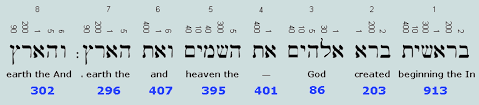There is also a relationship between 2664 and the 2669 times the value (401) of the word Et (את), the 4th word and article that precedes H’Shamayim (השמים) as found in the Torah. While the difference between 2669 and 2664 is 5 like the 5th word, the complete numerical value of the word Et (את) is 401 + 23 = 424, that of Moshiach Ben David.  Another way to get to 2669, is utilizing the word for “The Annointed One, (המשיח)” which includes the hei(ה) of H’Shamayim (השמים) as well. As a final note on that matter, some look at the 95 (המים) in 395 (השמים), not just as the spiritual waters or hyperspace, but as “The King (המלך).” The king and the waters share a few mathematical properties in common, which is not so common for most words in the Torah. While both have the same gematria of 90 without the prefix “the” and 95 with it, both have not only the same number of letters, but both also share the same ordinal value of 36, or 41 with the prefix hei(ה). They also share the same small gematria value, (4+1+4) = (4+3+2) = 9 or alternatively (5+4+1+4) = (5+4+3+2) = 14. These properties hold true for any word comprised on the same exact root letters.
Now, let us look for more clues inside the Torah’s 5th word, H’Shamayim (השמים) of numerical value 395. It is simple arithmetic that 395 = (400 – 5), which equals (5 x 80) – 5, reminiscent of the Torah’s 80,000 – 52 words, 58 total elements, and 5845 verses.  As the Shin(ש) in (השמים) is 300, and the Mem(מם) is 80, the Hey(ה) is 5 and the Yud(י) is 10 or 4+3+2+1, we also have the 304,805 letters represented. Though more significant is the connection between the Mem(מם) as 80, the value of the letter Pe(פ), not just because of the 4805 Pes(פ), in the Torah, but because it is the same numerical value as the Hebrew word for Throne (כס).  Kes(כס) and Mem(מם) also share the same ordinal value, 26. Now the name of the letter Pe(פא) is spelled out with the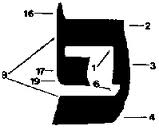letter alef(א), which is apropos since the word for throne, Kiseh(כסא), appears in the Torah with the additional alef(א). The clue for the alef(א) hidden within the energetic confines of the letter Pe(פ) is found in its spiraling design Pe(פ), which we know from the Arizal purposely conceals a letter Bet (ב).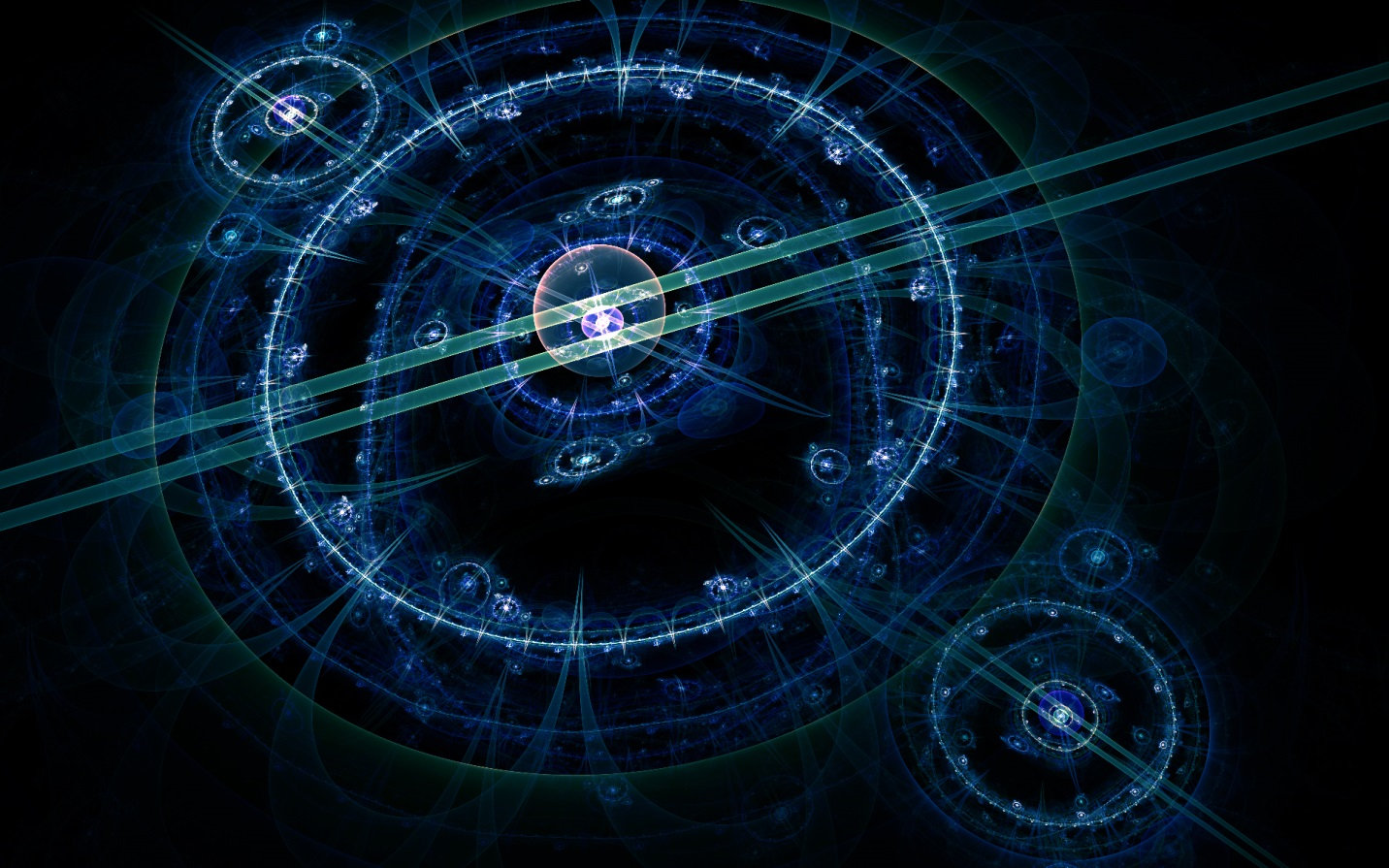As opposed to the pure math in the other sections, this section is all just conjecture. We are not saying that a mini-Moshiach is climbing into the 5th word of the Torah. Nevertheless, the space provided by the missing alef (א) in the 5th word allows for Moshiach to harness the multi-dimensional hypercube and engage the hyperdrive for us.  The Torah hints that Moses (משה) did it as well. The Name or Triplet Moshe (משה) in found in the 5th word H’Shamayim (השמים) and also as in the 5th triplet (מהש) of the 72 Triplets. The Torah tells us that Moshe (השמים) ascended Mt Sinai for 40 days (השמים) to receive the 10 (השמים) Commandments.  Is it odd that the Zohar would tell us that Moses received the 173 keys to Heaven (השמים) and that 173 is the 40th prime? Or is it Rabbi Shimon Bar Yochai understood what he was talking about 1900 years ago.  Neither primes, nor mathematics other than simple gematria is ever discussed in the Zohar, so he was not reverse engineering the word for Heaven, he was making legitimate energetic connections to it.  We are ones fortunate enough today to be able to partially reverse engineer it to graps a little of what he knew intuitively. Oddly, the small gematria of ‘the waters” (5*4*1*4) = 80 and “The King” (5*4*3*2) = 120, a possible reference to Moses’ 80 years prior to Mt Sinai, and his total 120-year lifespan, as promised by G-d in Genesis 6:3.
How do we know an alef (א) was consciously or purposefully missing? We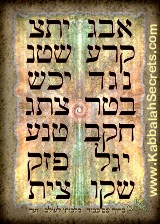can infer this from the relationship between the 42-Letter Name of numerical value 3701 and the first verse of numerical value 2701 in that 1/3701 = .0002702 which represents the additional alef (א).  It would seem that a place, or opportunity, was figuratively reserved for both Moshe and Moshiach.  Of course, the difference between Moshe and Moshiach is 358 – 345 = 13.  And in case you had not noticed, those 173 keys, or the small gematria of the 42-Letter Name, 173, is 3701 in reverse.
My teacher, Rav Brandwein of blessed memory, once explained to me, regarding the 42-Letter Name that it takes two drops from below to awaken one above, and while 3701 – 2701 = 1000, (2 x 173) – 345 (Moshe) = 1.

## Partitioning

What is all this telling us? What are partitions? They are combinations of elements.
When these elements are the letters in the words of the Torah, they are no longer simple number grouping.  All the great kabbalists throughout time decoded the Torah by permuting and recombining the letters, including Rav Abraham Abulafia in numerous texts, Rav Isaac Luria and Rabbi Chaim Vital in the Kitve Ari, Rabbi Shimon Bar Yochai in the Zohar, and Abraham Avinu in the Sefer Yetzirah. Partitioning is the first step, followed by combinatorics, then permutations, then alpha-numeric translation and evaluation through the gematria ciphers. As with all processes, the first step is always the most difficult. Whether it was partitioning the energy vibration levels and 11 dimensions (including time) in the creation of the universe; or partitioning the alef-bet, representative of those levels, into two sets of 11 letters; or then partitioning the Torah into 79,975 words, 5845 verses, 54 portions, 670 paragraphs, 187 chapters and the 5 Books, that first step eventually gave rise to infinite dimensionality in which every eventuality was originally conceived and which lay latent from the outset. That mouthful means “the end was in the beginning.” Just as choosing to walk in a door or walk past it will change every moment of your life from then on, so too the first split guided everything that followed.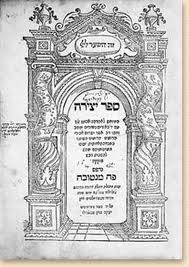We have already established that the 22 letters are elements to be partitioned. The Sefer Yetzirah calls them “building blocks,” which it breaks down into 3 Mothers, 7 Doubles, and 12 Elementals.” The Indices of the 7 known Bell Primes are 2, 3, 7, 13, 42, 55, 2841 and we have accounted for the numbers 13, 42 and 55 in terms of the Torah and the Shema prayer derived from it. Given unlimited efficiency, every number (and letter) would have at least one specific purpose; therefore, the numbers 2, 3 and 7 in the Bell Primes add up to 12, as in the 12 Elementals, especially given that 3 could represent the 3 Mothers and 7, the 7 Doubles.
Sefer Yetzirah 1:2
Ten Sefirot of Nothingness
And 22 Foundation Letters:
Three Mothers,
Seven Doubles
And Twelve Elementals
Of course, we would be remiss if we did not point out that 2 x 3 x 7 = 42. Or that 1/2 + 1/3 + 1/7 + 1/42 = 1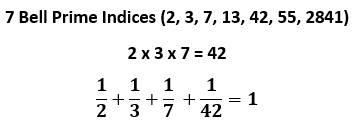As is becoming increasingly obvious, it is all about the One (1) and how to get there through the 42-Letter Name, the first verse of Bereshit and the Shema.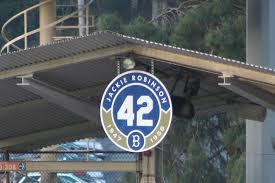Now go ask Google what is the answer to life, the universe and everything. The answer will always be 42. Regardless of why that is, do you think that it is just a cosmic coincidence? The end is in the beginning. Baseball is a game of numbers, so what number do you think hangs over every stadium? 42.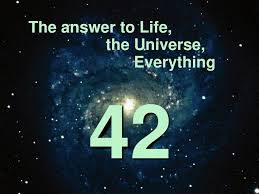Even the Name Google: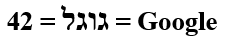In order to demonstrate the computational magnitude, enormity, and the rarity of the 7 Bell Prime Indices (2, 3, 7, 13, 42, 55, 2841), we will give you an idea what they actually represent. Each number corresponds to their respective Bell Prime (2, 5, 877, 27644437, 35742549198872617291353508656626642567, and 359334085968622831041960188598043661065388726959079837) and that only takes us to 55.  You can imagine what kind of enormous number corresponds to the 2841st index.
Given all that, as my colleague Peter recently pointed out, the first six Bell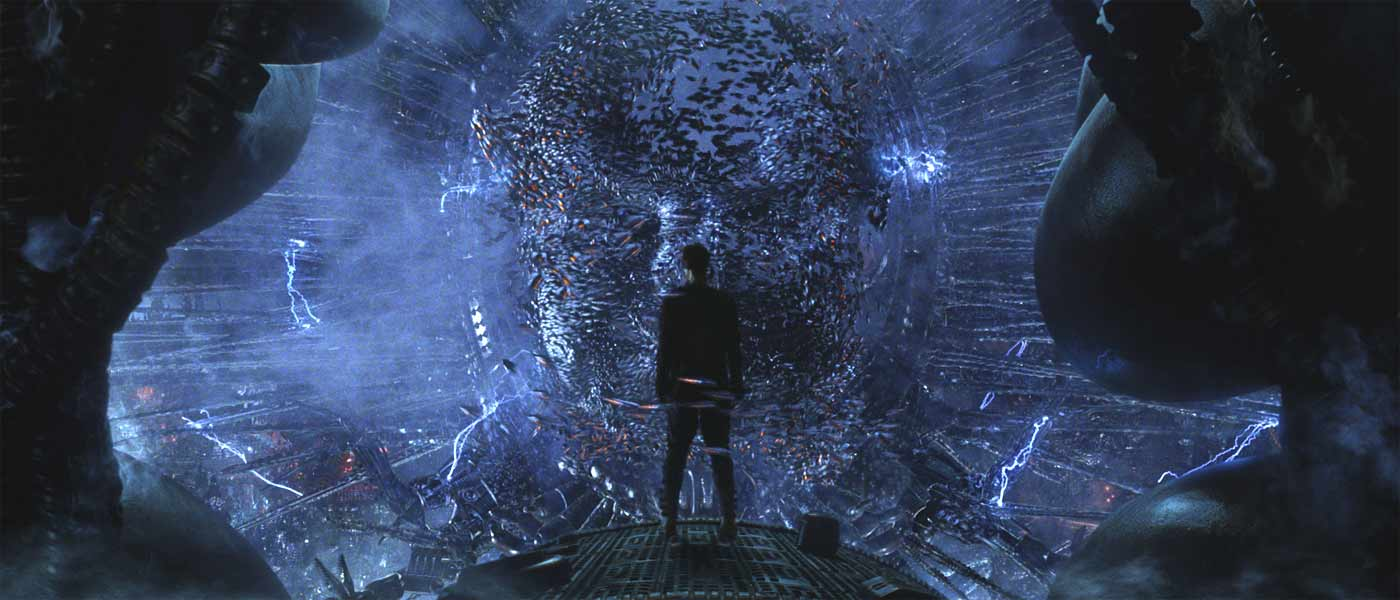Prime Indices (2 + 3 + 7 + 13 + 42 + 55) add up to 122, which given the exceedingly strong (13,42,55) Shema connection, the sum 122 can be seen as analogous to the middle 122-word paragraph of the Shema that came straight out of Devarim 11:13-21. So at this point, the 248 words of the Shema are split into 6 words of 25 letters, like the 625 square root of the Torah’s main elements, the 42-word paragraph, the middle (2+3+7+13+42+55) word paragraph and the final 72-word one plus the 6 silent words. Out of the 248 words, the first verse and the first and last paragraphs together total (6+42+72) = 120 words. This once again figuratively connects Moshe and Abraham, and once again connects one crucial partitioning to another, the 22 verses of the Shema to the 22 letters of the Alef-bet.
Is it feasible to believe that any man, even Moses, could make these calculations? That he would do so just so that he could incorporate them into the Shema and Bereshit?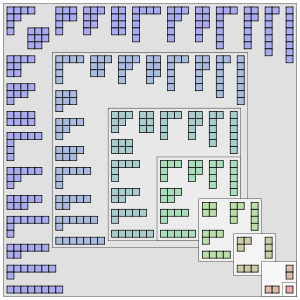Just in case we were to think this alignment was just a beautiful coincidence and not the work of a powerful supercomputer, or more likely, the infinite mind of the Creator, there are a few more facts we should mention.  First of all, there are exactly 42 ways to partition 10 elements, like the 10 sefirot or 10 dimensions. And 55 is both the sum of the integers from 1 to 10, and also the 10th Fibonacci Number, the number series based on the Phi proportion. Moreover, 13 is the 7th Fibonacci number and there are exactly 7 combinations of Fibonacci numbers within those first 10 Fibonacci numbers that sum to 42. As we will see later in this series, there are even more significant and singular ways the universe is connecting 10 and 42 and 1 and 42. If we were looking for a way to access the freedom of the 10 dimensional hyperspace, has the Torah and the universe given us enough clues yet?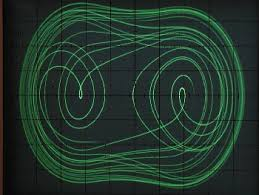In reviewing the Bell Prime Indices we see that 13 x 42 x 55 = 30030, or 10 x 3003, which is the sum of the gematria of the first 8 words (33 letters) of the Torah. It is also the sum of the integers from 1 -77 and there are 77 ways to partition the 12 Elementals. If we divide instead, we get 55/42/13 = .10073260073. Once again seeing an indicative key number (26) flanked by the number 73, meaning wisdom as previously explained. Last time, it was 314 (Shaddai); this time it is the Tetragrammaton (יהוה) of value 26, and of course the first verse has a value equal to the sum of the integers from 1 – 73.
Moreover, the triplet 600 in 55/42/13 = .10073260073 may represent the product of the digits in (1 x 3 x 4 x 2 x 5 x 5), and/or the 600 Egyptian chariots that chased the 600,000 Israelite men out of Egypt. If we sum those same digits we get (1+3+4+2+5+5) = 20, spiritually and kabbalistically analogous to Keter (כתר), the highest dimension, because the Hebrew word for 20, Esrim (עשרים), shares the same gematria of 620, which we also find by adding the results of our Product (600) and our sum (20).

(1 x 3 x 4 x 2 x 5 x 5) + (1 + 3 + 4 + 2 + 5 + 5) = 620 (כתר)

We mentioned earlier that all Hebrew words have triplet root letters and the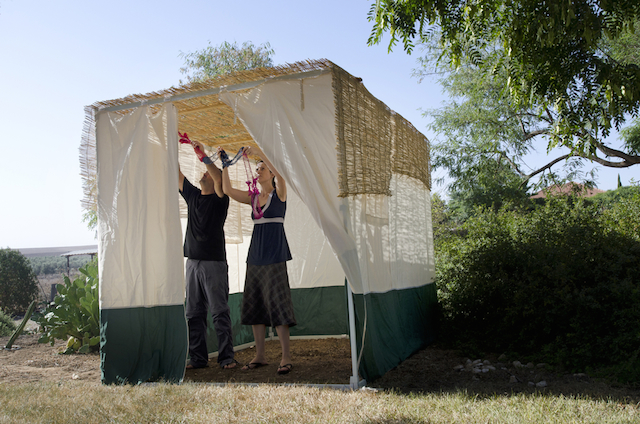root of Keter (כתר) is Kater (כתר), meaning surrounding, as in the endless surrounding the Tree-of-life in the center of the garden.  This is why the limit of the height of a sukkah in the holiday of Sukkot is 20 cubits, and why the 620 letters of the 10 Commandments was in chapter 20 of Exodus, and also why there are 62 letter Yuds(י) of numerical value 10 each within those 10 Commandments. Moreover the 10 Commandments begin at the 107,007th letter in the Torah, perhaps reminiscent to .10073260073, and they end at the 62nd paragraph.  Intelligent design!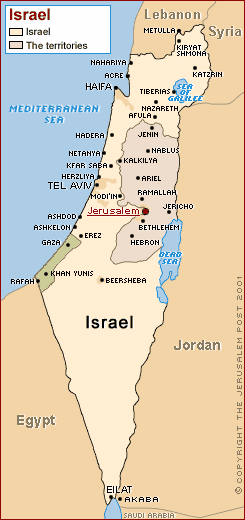As for a little contextual symmetry that steps beyond the Torah and into our world today. With its present borders, including the West Bank, East Jerusalem and the Golan Heights, Israel is exactly 11,466 square miles, which when converted to time, is 54 minutes shy of precisely 8 days, the time necessary for a covenant (brit). In the 54th paragraph of the Book of Shemot, we find the 10 Commandments at letter # 107,007 and chapter 70 in the Torah. The square root of those specific square miles ties right in, as if it were preplanned thousands of years ago: √11466 = 107.07.
We showed the integration of 6 of the 7 Bell Prime Indices (2, 3, 7, 13, 42, 55, 2841), but what of the 7th Bell Prime Index (2841) and how does it relate to the first 7-word verse of the Torah beside the obvious 28 letters? In our introduction article to this series we let it be known that we would be integrating and aligning the Torah’s first verse, the Shema’s first verse and the 42-Letter Name as the three entry portals to the world beyond (Olam Haba, the sea of Binah, hyperspace)–emergency escape valves if you will.  We saw how the gematria of each respectively added up to 2701 + 1118 + 3701 = 7520 so that they can be split in two to equal the pivot point on the Divine Calendar: 3760 HC (0 CE)
We should note here that 376 is the gematria of the most familiar Hebrew word, Shalom (שלום), meaning Peace. It also means Hello!
We also saw how the 6th of these elements, 55, corresponded with the 6th Bell number, (1,2,5,15,52 203, 877…) which was the sum of the first 3 letters of the Torah, etc.
If we pair up and add the 7th Bell Prime Index 2841 with the 7th Bell Number (877), we get (2841 + 877) = 3718, and 3718 + 42 = 3760 (0 CE).
In reflection of the 7th Bell and the number 42, if we look at the number 42 as the 4th root of 2, we get: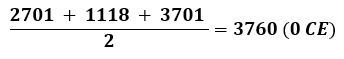Earlier, we applied the gematria cipher of the “product/sum” to the total gematria of the Torah. Applying it to the loose relationship between 2841 and 877 gives us (2841 x 877)/(2841+877) = 670.13, as in the 670 paragraphs in the Torah, and 13 once again. One notable aspect of this is that there are 13 digits in the 7 Bell Primes and 13 digits in the 7 Bell Prime Indices. And 13 digits in the 7 first elements of the Primeth Recurrence series.  In the next article in this series we will see just what an integral roll the number 13 plays. Is it a coincidence the Torah’s Creator built it into the Torah’s very first word, bereshit of numerical value 913? Is it a coincidence certain dark forces told the world that 13 was unlucky and that it should be erased from our buildings and elevators.
Another oddity about these 7 unique Bell Prime Indices (2, 3, 7, 13, 42, 55, 2841) is that their digits when partitioned as |2, 3, (2+3), (7+1+3), (4+2+5+5+2+8+4+1)| = |2, 3, 5, 11, 31| matching the Primeth Recurrence series (2, 3, 5, 11, 31…) perfectly. Einstein said, “God doesn’t play dice with the universe.” If He does, they are loaded.
Meanwhile, the sum of the digits in the 7 Bell Numbers is (1+2+5+1+5+5+2+2+0+3+8+7+7) is 48, as in the sum of the cubic lengths of all 7 Words in the first verse 48.778. Go figure!
And just like the 6 Bell Number digits that equaled 26, so too do the 6 Primeth Recurrence series digits (2+3+5+1+1+3+1+1+2+7). It is like someone is putting their fingerprint on all these rare “naturally occurring series” and all the equations that result from their interactions. The Tetragrammaton (יהוה) = 26, just like the 26 digits in the 7 elements of each of the two Bell Primes series combined.
It is as if you were in a lab and mixing whatever odd chemicals you could find in random ways and every time the reaction gave you similar results, the exact same amount of free energy.

## Crisscross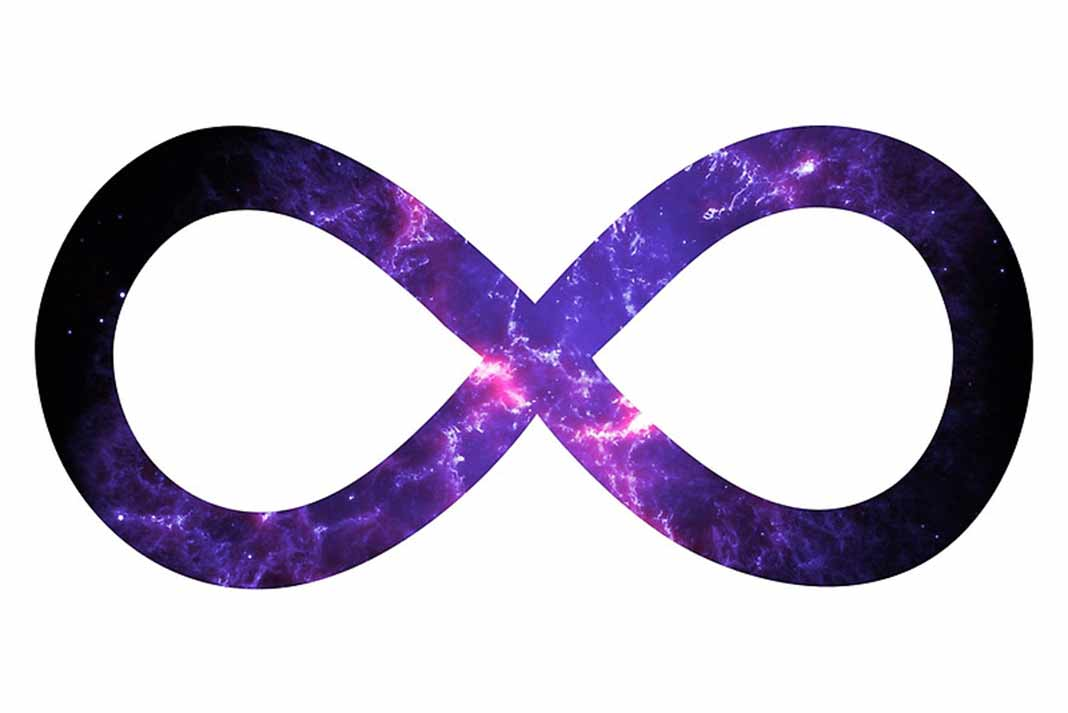We have found several instances so far in which the mathematics integrated into the Torah’s first 7 words and first 8 words have significance far beyond these verses. Yet another example is found in the simple division of the sum of the 8 words by the sum of the 7 words or 3003/2701 = 1.1118, giving us One(1) and 1118, the value of the Shema’s first verse.
Yet again, the Shema and the Torah’s first verse are integrated.
Moreover, there are 7 words and 8 words, 28 letters and 33 letters in the two verses respectively; their sum of (7 + 8 + 28 + 33) = 76, the ordinal value of the Torah’s first word (Bereshit).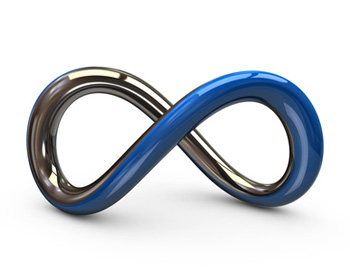We also see that the 7 words of the Torah’s first verse total 2701, which is the sum of integers from 1 – 73. Divide that by its words and letters, 2701/(7 +28) = 77.17… Then juxtapose that with the value of the 8 first words of the Torah, 3003, which is the sum of integers from 1 – 77. Divide that by its words and verses, 3003/(8 +33) = 73.24… Thus, the results of these two simple equations, 77 for the 7 words and 73 for the 8 words, reverse or crisscross their given integer sums: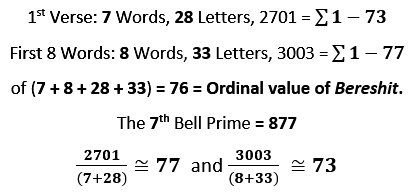Is it possible the 7th Bell Prime 877 was sending us a message from deep within the heart of the universe?Most of the time, the Torah’s Creator highlights things through redundancy yet sometimes He highlights things by omission. In the Torah we have the letters and the blank parchment they are written on. This same attribute applies to the universe as well.  The Kabbalists call this black fire and white fire. Certainly, this applies in the Torah, where the highest word value is 1500 and lowest 3, bounding the set of Torah word values and limiting it to 1498 possibilities, matching the value of the combined 1st, 2nd, 3rd, and 7th words of the Torah’s first verse (913, 203, 86, and 296).  And since there are 1024 actual word values used, (1498 – 1024) possible values are not utilized, making the size of this empty set, 474, as in the 11th dimension (sefira), Da’at or Knowledge. Given the 1024 vertices of the 10-dimensional cube, it is possible that there are somehow also 474 virtual vertices containing hidden knowledge.
As for white fire of hidden knowledge in the universe itself, there is only one positive integer under 100 that man and his computers have not been able to find a solution to the Diophantine equation (a3 + b3 + c3) that do not comply with for technical reasons either (9K + 4) or (9K +5). So far, they have checked all the possibilities through all the combinations through 1016 digits. That number: 42.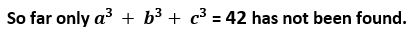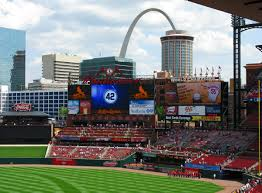And if you recall, dividing the number 9 by the numerical string formed by the 42-Letter Name of G-d gave us 9/.1230490… = 73.141593 or 70 + Pi. Was that extraordinary equation hinted at, alluded to, or integrated with the cubic length of the “bereshit:9.70?

Things are starting to take shape, albeit one that is somewhat beyond our comprehension. Nevertheless, we were never meant to figure it all out, just to understand enough to make contact.  In the next article, everything comes full circle, literally.
Have an amazing Shabbat and Shavuot and make sure you make the highest connections possible.
Ezra